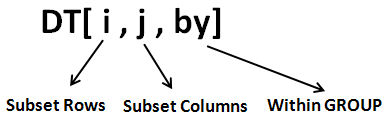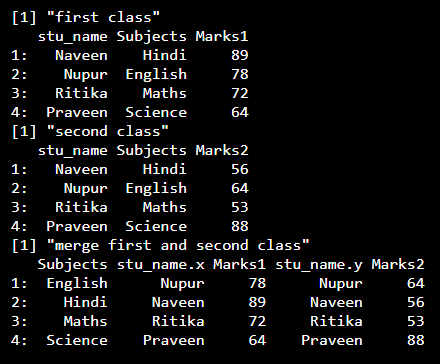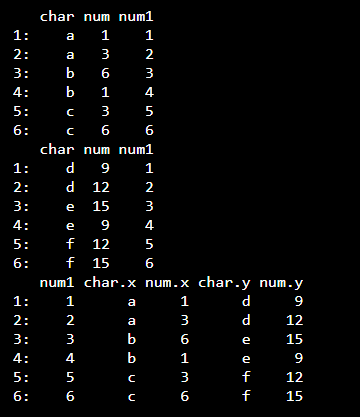Open in App
Not now

# Merge Two data.table Objects in R

• Last Updated : 23 Aug, 2021

data.table is a package that is used for working with tabular data in R. It provides an enhanced version of “data.frames”, which are the standard data structure for storing data in base R.

## Installation

Installing “data.table” package is no different from other R packages. Its recommended to run “install.packages()” to get the latest version on the CRAN repository.

Syntax:

`install.packages('data.table')`

The syntax of data.table is shown in the image below :• The first parameter of “data.table” i refers to rows. It implies subsetting rows.
• The second parameter of “data.table” j refers to columns. It implies subsetting columns (dropping / keeping).
• The third parameter of “data.table” by refers to adding a group so that all calculations would be done within a group.

For merging the same syntax is used except it is preceded by merge.

Syntax:

merge.data.table

Example: R program to merge two data.table objects

## R

library(“data.table”)

print(“first class”)

# Create first data.table
class1 <- data.table(stu_name = c('Naveen','Nupur','Ritika','Praveen'), Subjects = c('Hindi','English','Maths','Science'), Marks1 = c(89,78,72,64)) # Print first data.table print(class1) print("second class") # Create second data.table class2 <- data.table(stu_name = c('Naveen','Nupur','Ritika','Praveen'), Subjects = c('Hindi','English','Maths','Science'), Marks2 = c(56,64,53,88)) # Print second data.table print(class2) print("merge first and second class") # Merge data.tables merge_class <- merge.data.table(class1, class2, by.x = "Subjects", by.y = "Subjects") # Print merged data.table print(merge_class)

Output:Example: R program to merge two data.table objects

## R

library(“data.table”)

# table 1
D1 = data.table(char=rep(c(“a”,”b”,”c”),each=2),
num=c(1,3,6), num1=1:6)
D1

# table 2
D2 = data.table(char=rep(c(“d”,”e”,”f”),each=2),
num=c(9,12,15), num1=1:6)
D2

# merge table
D3 = merge.data.table(D1,D2, by.x=”num1″, by.y=”num1″)
D3

Output:My Personal Notes arrow_drop_up